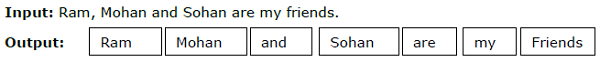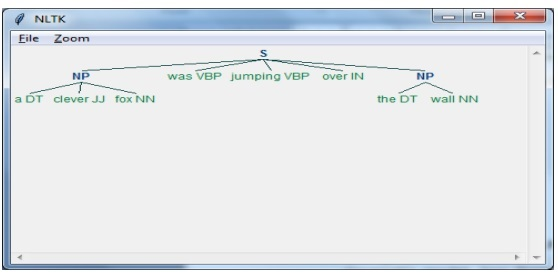# Python Web Scraping - Dealing with Text

In the previous chapter, we have seen how to deal with videos and images that we obtain as a part of web scraping content. In this chapter we are going to deal with text analysis by using Python library and will learn about this in detail.

## Introduction

You can perform text analysis in by using Python library called Natural Language Tool Kit (NLTK). Before proceeding into the concepts of NLTK, let us understand the relation between text analysis and web scraping.

Analyzing the words in the text can lead us to know about which words are important, which words are unusual, how words are grouped. This analysis eases the task of web scraping.

## Getting started with NLTK

The Natural language toolkit (NLTK) is collection of Python libraries which is designed especially for identifying and tagging parts of speech found in the text of natural language like English.

### Installing NLTK

You can use the following command to install NLTK in Python −

```pip install nltk
```

If you are using Anaconda, then a conda package for NLTK can be built by using the following command −

```conda install -c anaconda nltk
```

After installing NLTK, we have to download preset text repositories. But before downloading text preset repositories, we need to import NLTK with the help of import command as follows −

```mport nltk
```

Now, with the help of following command NLTK data can be downloaded −

```nltk.download()
```

Installation of all available packages of NLTK will take some time, but it is always recommended to install all the packages.

## Installing Other Necessary packages

We also need some other Python packages like gensim and pattern for doing text analysis as well as building building natural language processing applications by using NLTK.

gensim − A robust semantic modeling library which is useful for many applications. It can be installed by the following command −

```pip install gensim
```

pattern − Used to make gensim package work properly. It can be installed by the following command −

```pip install pattern
```

## Tokenization

The Process of breaking the given text, into the smaller units called tokens, is called tokenization. These tokens can be the words, numbers or punctuation marks. It is also called word segmentation.

### ExampleNLTK module provides different packages for tokenization. We can use these packages as per our requirement. Some of the packages are described here −

sent_tokenize package − This package will divide the input text into sentences. You can use the following command to import this package −

```from nltk.tokenize import sent_tokenize
```

word_tokenize package − This package will divide the input text into words. You can use the following command to import this package −

```from nltk.tokenize import word_tokenize
```

WordPunctTokenizer package − This package will divide the input text as well as the punctuation marks into words. You can use the following command to import this package −

```from nltk.tokenize import WordPuncttokenizer
```

## Stemming

In any language, there are different forms of a words. A language includes lots of variations due to the grammatical reasons. For example, consider the words democracy, democratic, and democratization. For machine learning as well as for web scraping projects, it is important for machines to understand that these different words have the same base form. Hence we can say that it can be useful to extract the base forms of the words while analyzing the text.

This can be achieved by stemming which may be defined as the heuristic process of extracting the base forms of the words by chopping off the ends of words.

NLTK module provides different packages for stemming. We can use these packages as per our requirement. Some of these packages are described here −

PorterStemmer package − Porter’s algorithm is used by this Python stemming package to extract the base form. You can use the following command to import this package −

```from nltk.stem.porter import PorterStemmer
```

For example, after giving the word ‘writing’ as the input to this stemmer, the output would be the word ‘write’ after stemming.

LancasterStemmer package − Lancaster’s algorithm is used by this Python stemming package to extract the base form. You can use the following command to import this package −

```from nltk.stem.lancaster import LancasterStemmer
```

For example, after giving the word ‘writing’ as the input to this stemmer then the output would be the word ‘writ’ after stemming.

SnowballStemmer package − Snowball’s algorithm is used by this Python stemming package to extract the base form. You can use the following command to import this package −

```from nltk.stem.snowball import SnowballStemmer
```

For example, after giving the word ‘writing’ as the input to this stemmer then the output would be the word ‘write’ after stemming.

## Lemmatization

An other way to extract the base form of words is by lemmatization, normally aiming to remove inflectional endings by using vocabulary and morphological analysis. The base form of any word after lemmatization is called lemma.

NLTK module provides following packages for lemmatization −

WordNetLemmatizer package − It will extract the base form of the word depending upon whether it is used as noun as a verb. You can use the following command to import this package −

```from nltk.stem import WordNetLemmatizer
```

## Chunking

Chunking, which means dividing the data into small chunks, is one of the important processes in natural language processing to identify the parts of speech and short phrases like noun phrases. Chunking is to do the labeling of tokens. We can get the structure of the sentence with the help of chunking process.

### Example

In this example, we are going to implement Noun-Phrase chunking by using NLTK Python module. NP chunking is a category of chunking which will find the noun phrases chunks in the sentence.

### Steps for implementing noun phrase chunking

We need to follow the steps given below for implementing noun-phrase chunking −

### Step 1 − Chunk grammar definition

In the first step we will define the grammar for chunking. It would consist of the rules which we need to follow.

### Step 2 − Chunk parser creation

Now, we will create a chunk parser. It would parse the grammar and give the output.

### Step 3 − The Output

In this last step, the output would be produced in a tree format.

First, we need to import the NLTK package as follows −

```import nltk
```

Next, we need to define the sentence. Here DT: the determinant, VBP: the verb, JJ: the adjective, IN: the preposition and NN: the noun.

```sentence = [("a", "DT"),("clever","JJ"),("fox","NN"),("was","VBP"),("jumping","VBP"),("over","IN"),("the","DT"),("wall","NN")]
```

Next, we are giving the grammar in the form of regular expression.

```grammar = "NP:{<DT>?<JJ>*<NN>}"
```

Now, next line of code will define a parser for parsing the grammar.

```parser_chunking = nltk.RegexpParser(grammar)
```

Now, the parser will parse the sentence.

```parser_chunking.parse(sentence)
```

Next, we are giving our output in the variable.

```Output = parser_chunking.parse(sentence)
```

With the help of following code, we can draw our output in the form of a tree as shown below.

```output.draw()
```## Bag of Word (BoW) Model Extracting and converting the Text into Numeric Form

Bag of Word (BoW), a useful model in natural language processing, is basically used to extract the features from text. After extracting the features from the text, it can be used in modeling in machine learning algorithms because raw data cannot be used in ML applications.

### Working of BoW Model

Initially, model extracts a vocabulary from all the words in the document. Later, using a document term matrix, it would build a model. In this way, BoW model represents the document as a bag of words only and the order or structure is discarded.

### Example

Suppose we have the following two sentences −

Sentence1 − This is an example of Bag of Words model.

Sentence2 − We can extract features by using Bag of Words model.

Now, by considering these two sentences, we have the following 14 distinct words −

• This
• is
• an
• example
• bag
• of
• words
• model
• we
• can
• extract
• features
• by
• using

## Building a Bag of Words Model in NLTK

Let us look into the following Python script which will build a BoW model in NLTK.

First, import the following package −

```from sklearn.feature_extraction.text import CountVectorizer
```

Next, define the set of sentences −

```Sentences=['This is an example of Bag of Words model.', ' We can extract
features by using Bag of Words model.']
vector_count = CountVectorizer()
features_text = vector_count.fit_transform(Sentences).todense()
print(vector_count.vocabulary_)
```

### Output

It shows that we have 14 distinct words in the above two sentences −

```{
'this': 10, 'is': 7, 'an': 0, 'example': 4, 'of': 9,
'bag': 1, 'words': 13, 'model': 8, 'we': 12, 'can': 3,
'extract': 5, 'features': 6, 'by': 2, 'using':11
}
```

## Topic Modeling: Identifying Patterns in Text Data

Generally documents are grouped into topics and topic modeling is a technique to identify the patterns in a text that corresponds to a particular topic. In other words, topic modeling is used to uncover abstract themes or hidden structure in a given set of documents.

You can use topic modeling in following scenarios −

### Text Classification

Classification can be improved by topic modeling because it groups similar words together rather than using each word separately as a feature.

### Recommender Systems

We can build recommender systems by using similarity measures.

## Topic Modeling Algorithms

We can implement topic modeling by using the following algorithms −

Latent Dirichlet Allocation(LDA) − It is one of the most popular algorithm that uses the probabilistic graphical models for implementing topic modeling.

Latent Semantic Analysis(LDA) or Latent Semantic Indexing(LSI) − It is based upon Linear Algebra and uses the concept of SVD (Singular Value Decomposition) on document term matrix.

Non-Negative Matrix Factorization (NMF) − It is also based upon Linear Algebra as like LDA.

The above mentioned algorithms would have the following elements −

• Number of topics: Parameter
• Document-Word Matrix: Input
• WTM (Word Topic Matrix) & TDM (Topic Document Matrix): Output